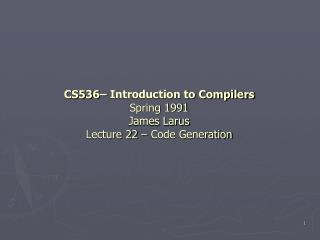DownloadDownload PresentationCS536– Introduction to Compilers Spring 1991 James Larus Lecture 22 – Code Generation

# CS536– Introduction to Compilers Spring 1991 James Larus Lecture 22 – Code Generation

Télécharger la présentation## CS536– Introduction to Compilers Spring 1991 James Larus Lecture 22 – Code Generation

- - - - - - - - - - - - - - - - - - - - - - - - - - - E N D - - - - - - - - - - - - - - - - - - - - - - - - - - -
##### Presentation Transcript

1. CS536– Introduction to CompilersSpring 1991James LarusLecture 22 – Code Generation

2. Code Generation • Process of producing assembly code from intermediate representation (IR) • Final stage of most compilers • Two aspects • Decide what code to produce for a language construct • Decide how to generate it from parse tree • General idea • Do a pre-order/post-order traversal of parse tree • At each node, generate code to evaluate operands • Generate code for node that uses operands

3. Example: Arithmetic Expressions + x • Use the stack to evaluate expressions • e.g x+2*y • gen_plus (AST *n) { generate (n->left); generate (n->right); gen (“ lw st0, 0(\$sp)” ); /* Pop right operand */ gen ("lw st1, 4(\$sp)"); /* Pop left */ gen ("addiu \$sp, \$sp, 8"); gen ("addu \$t3, \$t0, \$t1"); /* Add operands */ gen ("addu \$sp, \$sp, -4"); /* Push sum */ gen ("sw \$t3, 4(\$sp)"); } • gen_var (AST *n) { gen ("lw \$t0, %d(\$fp) ", stack_offset (n0); gen("addiu \$sp, \$sp, -4"); gen ("sw \$t0, 4(\$sp) "); } var int var x y 2

4. Example, cont’d + • gen_plus(+) • gen_var(x) lw \$t0, 4(\$fp) addiu \$sp, \$sp, -4 sw \$t0, 4(\$sp) • gen_times(*) • gen_lit(2) li \$t0, 2 addiu \$sp, \$sp, -4 sw \$t0, 4(\$sp) • gen_var (y) lw \$t0, 8(\$fp) addiu \$sp, \$sp, -4 sw \$t0, 4(\$sp) • lw \$t0, 0(\$sp) lw \$t1, 4(\$sp) addiu \$sp, \$sp, 8 addu \$t3, \$t0, \$t1 addu \$sp, \$sp, -4 sw \$t3, 4(\$sp) x var int var x y 2

5. Example, cont’d • Terrible code • Doesn’t make effective use of machine registers or instructions • Contains many redundant moves • Easy to generate • Each code generation routine operates in isolation • Produce code assuming operands are on stack and leave result on stack • Alternative is to worry where operands should be placed and where they are stored • Easy to extend stack-based code to rest of language

6. Generation for Statements • Assignment statements • gen_assign (AST *n) { generate (n->expr); pop ("\$t0"); gen ("sw t0, %d(\$fp)", offset (n->var)); } • If statement • Evaluate predicate expression • If result is false, jump to alternative code • Evaluate consequent • Jump to end of statement • Evaluate alternative

7. If Statements, cont’d • gen_if_then_else (AST *n) { altern_label =make_label (); and_label =make_label (); generate (n->predicate); pop ("\$t0"); gen ("beqz \$t0, %s”, altern_label); generate (n->consequent); gen ("j %s", end_label); gen ("5s:", altern_label); generate (n->alternative); gen ("%s:", end_label); }

8. Example: If Statement • If x = 2 then y := 1 else y := 2; lw \$t0, 4(\$fp) addiu \$sp, \$sp, -4 sw \$t0, 4(\$sp) push (2) lw \$t0, 8(\$sp) lw \$t1, 4(\$sp) addiu \$sp, \$sp, 8 seq \$t2, \$t0, \$t1 push (\$t2) pop (\$t0) beqz \$t0,L1 push (1) pop (\$t0) sw \$t0, 8(\$fp) I L2 ….

9. Example, cont’d • Real compiler would eliminate push/pop and keep values in registers • li \$t0, 2 lw \$t1, 4(\$fp) seq \$t2, \$t0, \$t1 beqz \$t2, L1 li \$t0, l sw \$t0, 8(\$fp) j L2 L1: li \$t0, 2 sw \$t0, 8(\$fp) L2: • Evaluate predicate for control, not value • li \$t0, 2 lw \$t1, 4(\$fp) bneq \$t0, \$t1, L1 …..

10. Evaluation for Control • Many machined do not have comparison instructions that produce values • Only have compare-and-branch instructions • To produce value, generate sequence of instructions cmp_br_eq \$t0, \$t1, L3 push (0) j L4 L3: push (1) L4: • Need more complex generation routine • Generate_for-control (AST *n, char *true_label, char *false_label0 • Becomes complex for elaborate expressions

11. Code Generation for Loops • while predicate do body od • Produced code to evaluate predicate • If predicate is false jump to end of loop • Evaluate body • Jump to 1 • for i := n to m do body od • Evaluate lower bound and save in I • Evaluate upper bound and save in tmp • Evaluate predicate I <= tmp • If predicate is false, jump to end of loop • Evaluate body • Jump to 3